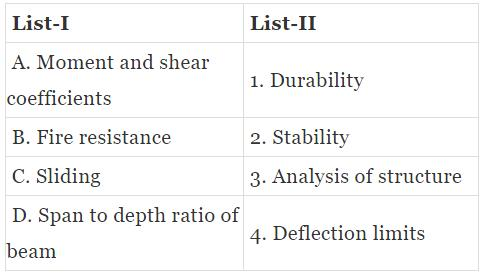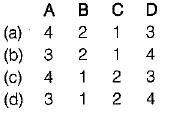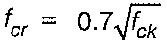Courses

# Miscellaneous - 2

## 10 Questions MCQ Test Topicwise Question Bank for GATE Civil Engineering | Miscellaneous - 2

Description
This mock test of Miscellaneous - 2 for Civil Engineering (CE) helps you for every Civil Engineering (CE) entrance exam. This contains 10 Multiple Choice Questions for Civil Engineering (CE) Miscellaneous - 2 (mcq) to study with solutions a complete question bank. The solved questions answers in this Miscellaneous - 2 quiz give you a good mix of easy questions and tough questions. Civil Engineering (CE) students definitely take this Miscellaneous - 2 exercise for a better result in the exam. You can find other Miscellaneous - 2 extra questions, long questions & short questions for Civil Engineering (CE) on EduRev as well by searching above.
QUESTION: 1

Solution:
QUESTION: 2

Solution:
QUESTION: 3

### Match List-I with List-ll and select the correct answer using the codes given below the lists:Codes:Solution:
QUESTION: 4

Diagonal tension in a beam

Solution:
QUESTION: 5

The basic assumption of plane sections normal to the neutral axis before bending, remaining plane and normal to the neutral axis after bending, leads to

Solution:
QUESTION: 6

The modulus of rupture of concrete gives

Solution:

Modulus of rupture as obtained by performing flexure test on concrete gives the theoretical maximum tensile stress on concrete specimen.

QUESTION: 7

The characteristic strength of concrete is defined as that compressive strength below which not more than

Solution:
QUESTION: 8

Consider the following statements:
1. Maximum strain in concrete at the outermost compression fibre is taken to be 0.0035 in bending.
2. The maximum compressive strain in concrete in axial compression is taken as 0.002.
Keeping the provisions of IS : 456-2000 on limit state design in mind, which of the following is true?

Solution:
QUESTION: 9

To determine the modulus of rupture, the size of test specimen used is

Solution:

The modulus of rupture test employs a 150 x 150 x 700 mm Plain concrete beam. The beam is simply supported and subjected to third-points loading until failure. Assuming a linear stress distribution across the cross-section, the theoretical maximum tensile stress reached in the extreme fibre is termed the modulus of rupture (fcr). It is obtained by applying the flexure formula,
fcr = M/Z
Modulus of rupture is given by,QUESTION: 10

For walls, columns and vertical faces of all structural members, the form work is generally removed after

Solution: